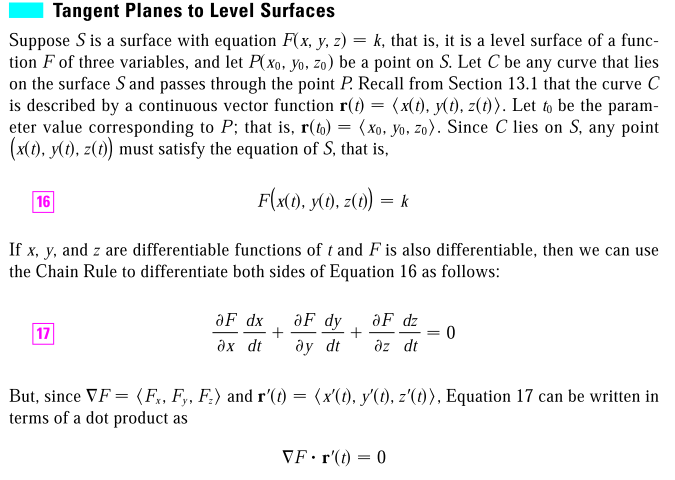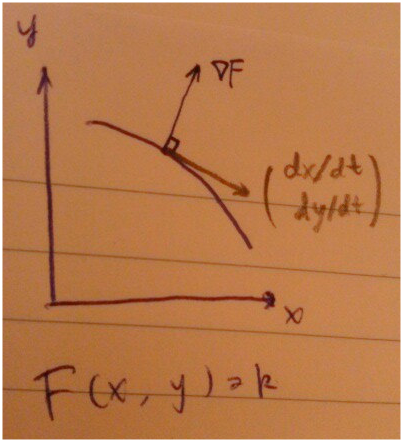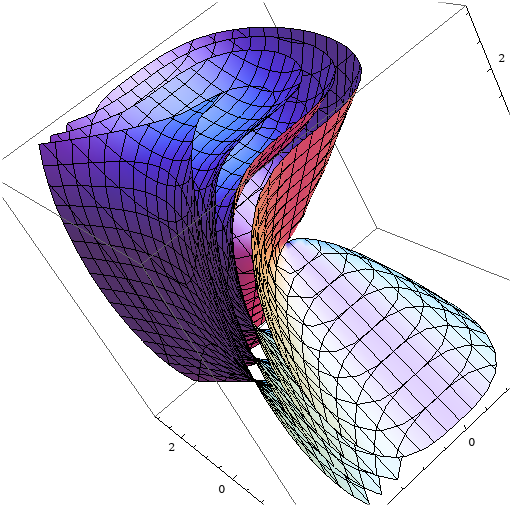## Gradient vector is perpendicular to the tangent plane

Gradient vector is perpendicular to the tangent planeThe main idea here is to setup a level surface, and find an arbitrary curve through one fix point on the surface, get the direction vector (which is tangent to the curve, and since it is tangent to any arbitrary curve on the surface, it must be tangent to the surface) of the curve at this point.

Then they show that the gradient vector is orthogonal to the tangent vector, since it is so for every such tangent vector, it must be orthogonal to the level surface.

The process can be simplified by reducing to 2D:Remember: The curve is a level curve, one contour line out of a contour plot. The insight is given by parameterize this contour line by \$t\$, then find out the relationship between the tagent vector and the contour by using the chain rule.

Visualization of level lines and surfaces by mathematica: